# NURBS: Important PropertiesGiven a set of n+1 control points P0, P1, ..., Pn, each of which is associated with a non-negative weight wi (i.e., Pi has weight wi >= 0), and a knot vector U = { u0, u1, ..., um } of m+1 knots, the NURBS curve of degree p is defined as follows: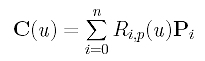where Ri,p(u) is defined as follows: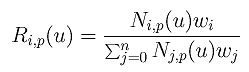The reason a different notation is used is because we want to rewrite the definition of a NURBS curve in a form as close to that of a B-spline curve as possible. In the above notation, Ri,p(u)'s are NURBS basis functions.### Important Properties of NURBS Basis Functions

Since NURBS is a generalization of B-spline, it should have all properties of B-splines. The following are some of the most important ones for NURBS basis functions. Please compare them with those of B-spline basis functions.
1. Ri,p(u) is a degree p rational function in u
2. Nonnegativity -- For all i and p, Ri,p(u) is nonnegative
3. Local Support -- Ri,p(u) is a non-zero on [ui,ui+p+1)
Since Ni,p(u) is non-zero on [ui,ui+p+1), so is Ri,p(u). Note that we assume wi's are non-negative.
4. On any knot span [ui, ui+1), at most p+1 degree p basis functions are non-zero, namely: Ri-p,p(u), Ri-p+1,p(u), Ri-p+2,p(u), ..., and Ri,p(u)
5. Partition of Unity -- The sum of all non-zero degree p basis functions on span [ui, ui+1) is 1:
6. If the number of knots is m+1, the degree of the basis functions is p, and the number of degree p basis functions is n+1, then m = n + p + 1 :
7. Basis function Ri,p(u) is a composite curve of degree p rational functions with joining points at knots in [ui, ui+p+1 )
8. At a knot of multiplicity k, basis function Ri,p(u) is Cp-k continuous.
Therefore, increasing multiplicity decreases the level of continuity, and increasing degree increases continuity.
9. If wi = c for all i, where c is a non-zero constant, Ri,p(u) = Ni,p(u)
Therefore, B-spline basis functions are special cases of NURBS basis functions when all weights become a non-zero constant. We have mentioned the special of c = 1.### Important Properties of NURBS Curves

The following lists important properties of NURBS curves. Please compare them with those of B-spline curves. Note that a NURBS can be open, clamped and closed. Like B-spline curves, if the first p+1 knots and the last p+1 knots are equal to the left end and right end of the domain, the curve is clamped.

1. NURBS curve C(u) is a piecewise curve with each component a degree p rational curve
Actually, each component is a rational Bézier curve.
2. Equality m = n + p + 1 must be satisfied
3. A clamped NURBS curve C(u) passes through the two end control points P0 and Pn
4. Strong Convex Hull Property: the NURBS curve is contained in the convex hull of its control points. Moreover, if u is in knot span [ui,ui+1), then C(u) is in the convex hull of control points Pi-p, Pi-p+1, ..., Pi
We have made it very clear that all weights must be non-negative. If some of them are negative, the strong convex hull property or even the convex hull property will not hold. In the following, the left figure is a NURBS curve of degree 2 with n = 2, m = 5 and the first three and last three knots clamped. The weights of the two control points at both ends are 1's and the weight of the middle control point is 0.5. This is actually an elliptic arc. The curve segment lies in the convex hull.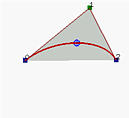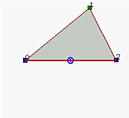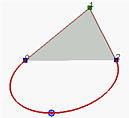The middle figure has the weight of the middle control point set to zero. Since this control point has no effect, the result is the line segment determined by the endpoints. It still lies in the convex hull.

If the weight is changed to -0.5, the curve segment is not contained in the convex hull and hence the convex hull property fails.

5. Local Modification Scheme: changing the position of control point Pi only affects the curve C(u) on interval [ui, ui+p+1)
This follows from the local modification scheme property of B-spline basis functions. Recall that Ri,p(u) is non-zero on interval [ui, ui+p+1). If u is not in this interval, since Ri,p(u) is zero and Ri,p(u)Pi has no effect in computing p(u). On the other hand, if u is in the indicated interval, Ri,p(u) is non-zero, and if Ri,p(u)Pi is changed, so does C(u).

This local modification scheme is very important to curve design, because we can modify a curve locally without changing the shape in a global way. Moreover, if fine-tuning curve shape is required, one can insert more knots (and therefore more control points) so that the affected region could be restricted to a very narrow one. We shall talk about knot insertion later.

6. C(u) is Cp-k continuous at a knot of multiplicity k
If u is not a knot, C(u) is in the middle of a curve segment of degree p and is therefore infinitely differentiable. If u is a knot in the non-zero domain of Ri,p(u), since Ri,p(u) is only Cp-k continuous, so does C(u).
7. Variation Diminishing Property:
The variation diminishing property also holds for NURBS curves. If the curve is contained in a plane (resp., space), this means no straight line (resp., plane) intersects a NURBS curve more times than it intersects the curve's control polyline.
8. B-spline Curves and Bézier Curves Are Special Cases of NURBS Curves
If all weights are equal, a NURBS curve becomes a B-spline curve. If furthermore n = p (i.e., the degree of a B-spline curve is equal to n, the number of control points minus 1) and there are 2(p + 1) = 2(n + 1) knots with p + 1 of them clamped at each end, this NURBS curve reduces to a Bézier curve.
9. Projective Invariance
If a projective transformation is applied to a NURBS curve, the result can be constructed from the projective images of its control points. This is a nice property. When we want to apply a geometric or even projective transformation to a NURBS curve, this property guarantees that we can apply the transformation to control points and the transformed NURBS curve is defined by the transformed control points. Therefore, we do not have to transform the curve.

Note that Bézier curves and B-spline curves only satisfy the affine invariance property rather than this projective invariance property. This is because only NURBS curves involve projective transformations.